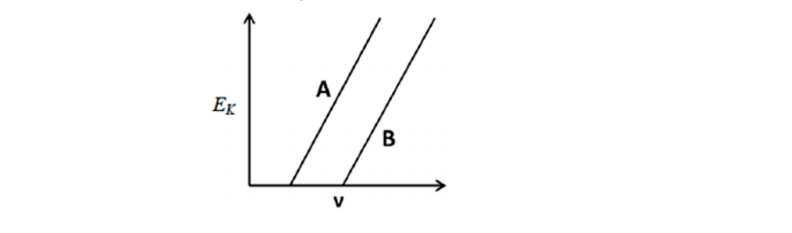# Problem: The figure below shows Ek plotted as a function of v for photoelectric emission from two metals, A and B. Which of the following statements for the two metals is correct? A. Both metals will produce the approximately equal currents under identical conditions. B. The threshold frequencies suggest that metal A is an alkali metal. C. The threshold frequency is the same for both metals. D. The threshold frequency of A is greater than that of B. E. The threshold frequency of A is less than that of B.

###### FREE Expert Solution
97% (463 ratings)
###### Problem Details

The figure below shows Ek plotted as a function of v for photoelectric emission from two metals, A and B. Which of the following statements for the two metals is correct?

A. Both metals will produce the approximately equal currents under identical conditions.

B. The threshold frequencies suggest that metal A is an alkali metal.

C. The threshold frequency is the same for both metals.

D. The threshold frequency of A is greater than that of B.

E. The threshold frequency of A is less than that of B.Frequently Asked Questions

What scientific concept do you need to know in order to solve this problem?

Our tutors have indicated that to solve this problem you will need to apply the Photoelectric Effect concept. You can view video lessons to learn Photoelectric Effect. Or if you need more Photoelectric Effect practice, you can also practice Photoelectric Effect practice problems.

What is the difficulty of this problem?

Our tutors rated the difficulty ofThe figure below shows Ek plotted as a function of v for pho...as medium difficulty.

How long does this problem take to solve?

Our expert Chemistry tutor, Jules took 3 minutes and 4 seconds to solve this problem. You can follow their steps in the video explanation above.